Theory and Modern Applications

# Variationally stable dynamic systems on time scales

## Abstract

In this paper we give a Lyapunov functional characterization of h-stability for nonlinear dynamic systems on time scales under the condition of ${u}_{\mathrm{\infty }}$-similarity between their variational systems. Furthermore, we give some examples related to the notions of ${u}_{\mathrm{\infty }}$-similarity and h-stability.

MSC:34N05, 39A30, 34D23, 34K20, 26E70.

## 1 Introduction

The theory on time scales has been developed as a generalization of both continuous and discrete time theory and applied to many different fields of mathematics [2, 3, 5, 6, 21, 23].

It is widely known that the various types of stability of nonlinear differential equations or difference equations can be characterized by using Lyapunov’s second method, the method of variation of parameters, and inequalities, etc. [1, 21, 22, 24, 38, 40].

Pinto  introduced the notion of h-stability for differential equations with the intention of obtaining results about stability for weakly stable differential systems under some perturbations. Also, Medina and Pinto  applied the h-stability to obtain a uniform treatment for the various stability notions in difference systems and extended the study of exponential stability to a variety of reasonable systems called h-systems. Pinto and Medina obtained the important properties about h-stability for the various differential systems and difference systems [2628, 30, 3335].

Choi et al.  investigated h-stability for the nonlinear differential systems by means of the notions of Lyapunov functions and ${t}_{\mathrm{\infty }}$-similarity introduced by Conti . Trench  introduced summable similarity as a discrete analog of Conti’s definition of ${t}_{\mathrm{\infty }}$-similarity and investigated the various stabilities of linear difference systems by using summable similarity. Choi and Koo  studied the variational stability for nonlinear difference systems by means of ${n}_{\mathrm{\infty }}$-similarity. Also, Choi et al. studied the asymptotic property and the h-stability of difference systems via discrete similarities and comparison principle . For detailed results about the various stabilities including the notions of h-stability and strong stability of dynamic systems on time scales, see [1218, 20, 25, 31].

In this paper we introduce the notion of ${u}_{\mathrm{\infty }}$-similarity which extends the continuous ${t}_{\mathrm{\infty }}$-similarity  and the discrete ${n}_{\mathrm{\infty }}$-summable similarity . Then we give a Lyapunov functional characterization of h-stability for nonlinear dynamic systems on time scales by assuming the condition of ${u}_{\mathrm{\infty }}$-similarity between its variational systems. Furthermore, we give some examples related to the notions of ${u}_{\mathrm{\infty }}$-similarity and h-stability on time scales.

## 2 Main results

We refer the reader to Ref.  for all the basic definitions and results on time scales necessary for this work (e.g., delta differentiability, rd-continuity, exponential function and its properties).

It is assumed throughout that a time scale $\mathbb{T}$ will be unbounded above. If $\mathbb{T}$ has a left-scattered maximum m, then ${\mathbb{T}}^{\kappa }=\mathbb{T}-\left\{m\right\}$. Otherwise, ${\mathbb{T}}^{\kappa }=\mathbb{T}$. Let ${\mathbb{R}}^{n}$ be the n-dimensional real Euclidean space. ${C}_{\mathrm{rd}}\left(\mathbb{T}×{\mathbb{R}}^{n},{\mathbb{R}}^{n}\right)$ denotes the set of all rd-continuous functions from $\mathbb{T}×{\mathbb{R}}^{n}$ to ${\mathbb{R}}^{n}$ and ${\mathbb{R}}_{+}=\left[0,\mathrm{\infty }\right)$.

We consider the dynamic system

${x}^{\mathrm{\Delta }}=f\left(t,x\right),\phantom{\rule{1em}{0ex}}x\left({t}_{0}\right)={x}_{0},{t}_{0}\in \mathbb{T},$
(2.1)

where $f\in {C}_{\mathrm{rd}}\left(\mathbb{T}×{\mathbb{R}}^{n},{\mathbb{R}}^{n}\right)$ with $f\left(t,0\right)=0$, and ${x}^{\mathrm{\Delta }}$ is the delta derivative of $x:\mathbb{T}\to {\mathbb{R}}^{n}$ with respect to $t\in \mathbb{T}$. We assume that ${f}_{x}=\frac{\partial f}{\partial x}$ exists and is rd-continuous on $\mathbb{T}×{\mathbb{R}}^{n}$. Let $x\left(t\right)=x\left(t,{t}_{0},{x}_{0}\right)$ be the unique solution of (2.1) satisfying the initial condition $x\left({t}_{0},{t}_{0},{x}_{0}\right)={x}_{0}$. For the existence and uniqueness of solutions of nonlinear dynamic system (2.1), see .

Also, we consider its associated variational systems

${v}^{\mathrm{\Delta }}={f}_{x}\left(t,0\right)v$
(2.2)

and

${z}^{\mathrm{\Delta }}={f}_{x}\left(t,x\left(t,{t}_{0},{x}_{0}\right)\right)z,$
(2.3)

where $I+\mu \left(t\right){f}_{x}\left(t,x\left(t\right)\right)$ is invertible for all $t\in \mathbb{T}$ and I denotes the $n×n$ identity matrix.

To establish our main results we will use the following lemmas.

Lemma 2.1 , Theorem 2.6.4]

Assume that $x\left(t,{t}_{0},{x}_{0}\right)$ and $x\left(t,{t}_{0},{y}_{0}\right)$ are the solutions of system (2.1) through $\left({t}_{0},{x}_{0}\right)$ and $\left({t}_{0},{y}_{0}\right)$ respectively, which exist for each $t\in {\mathbb{T}}_{0}$ and are such that ${x}_{0}$ and ${y}_{0}$ belong to a convex subset D of ${\mathbb{R}}^{n}$. Then

$x\left(t,{t}_{0},{y}_{0}\right)-x\left(t,{t}_{0},{x}_{0}\right)={\int }_{0}^{1}\mathrm{\Phi }\left(t,{t}_{0},{x}_{0}+\tau \left({y}_{0}-{x}_{0}\right)\right)\phantom{\rule{0.2em}{0ex}}d\tau \cdot \left({y}_{0}-{x}_{0}\right),\phantom{\rule{1em}{0ex}}t\in {\mathbb{T}}_{0},$

where Φ is a fundamental matrix of (2.3) and ${\mathbb{T}}_{0}=\mathbb{T}\cap \left[{t}_{0},\mathrm{\infty }\right)$.

This lemma can be proved in the same manner as that of Theorem 2.6.4 in , so we omit the detail.

Lemma 2.2 , Lemma 2.7.1]

Let $f\in {C}_{\mathrm{rd}}\left({\mathbb{T}}^{\kappa }×D,{\mathbb{R}}^{n}\right)$, where D is an open convex set in ${\mathbb{R}}^{n}$. Suppose that ${f}_{x}$ exists and is rd-continuous. Then

$f\left(t,{x}_{2}\right)-f\left(t,{x}_{1}\right)={\int }_{0}^{1}{f}_{x}\left(t,s{x}_{2}+\left(1-s\right){x}_{1}\right)\phantom{\rule{0.2em}{0ex}}ds\left({x}_{2}-{x}_{1}\right),\phantom{\rule{1em}{0ex}}t\in \mathbb{T}.$

In order to prove the variation of parameters formula on time scales, we need the following result on differentiability of solutions with respect to initial values.

Lemma 2.3 Assume that $f:{\mathbb{T}}_{0}×{\mathbb{R}}^{n}\to {\mathbb{R}}^{n}$ possesses partial derivatives on ${\mathbb{T}}_{0}×{\mathbb{R}}^{n}$ and ${f}_{x}\left(t,x\left(t,{t}_{0},{x}_{0}\right)\right)$ is rd-continuous on $\mathbb{T}$. Let $x\left(t\right)=x\left(t,{t}_{0},{x}_{0}\right)$ be the solution of (2.1), which exists for $t\ge {t}_{0}$ and let

$H\left(t,{t}_{0},{x}_{0}\right)=\frac{\partial f\left(t,x\left(t,{t}_{0},{x}_{0}\right)\right)}{\partial x}.$
(2.4)

Then

$\mathrm{\Phi }\left(t,{t}_{0},{x}_{0}\right)=\frac{\partial x\left(t,{t}_{0},{x}_{0}\right)}{\partial {x}_{0}}$
(2.5)

exists and is the solution of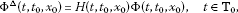(2.6)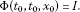(2.7)

The proof of Lemma 2.3 follows simply by differentiating the solution identity

${x}^{\mathrm{\Delta }}\left(t,{t}_{0},{x}_{0}\right)=f\left(t,x\left(t,{t}_{0},{x}_{0}\right)\right),\phantom{\rule{1em}{0ex}}t\ge {t}_{0},$

with respect to ${x}_{0}$. It is a special case of , Satz 1.2.22].

Remark 2.1 , Theorem 2.7.1]

$H\left(t,{t}_{0},{x}_{0}\right)$ in Lemma 2.3 is given by

$H\left(t,{t}_{0},{x}_{0}\right)=\underset{\xi \to \mathbf{0}}{lim}{\int }_{0}^{1}{f}_{x}\left(t,sx\left(t,{t}_{0},{x}_{0}\right)+\left(1-s\right)x\left(t,{t}_{0},{x}_{0}+\xi \right)\right)\phantom{\rule{0.2em}{0ex}}ds.$

It follows from Lemma 2.3 that the fundamental matrix solution $\mathrm{\Phi }\left(t,{t}_{0},0\right)$ of (2.2) is given by

$\mathrm{\Phi }\left(t,{t}_{0},0\right)=\frac{\partial x\left(t,{t}_{0},0\right)}{\partial {x}_{0}}$

and the fundamental matrix solution $\mathrm{\Phi }\left(t,{t}_{0},{x}_{0}\right)$ of (2.3) is given by

$\mathrm{\Phi }\left(t,{t}_{0},{x}_{0}\right)=\frac{\partial x\left(t,{t}_{0},{x}_{0}\right)}{\partial {x}_{0}}$

or equivalently

$x\left(t,{t}_{0},{x}_{0}\right)=\left[{\int }_{0}^{1}\mathrm{\Phi }\left(t,{t}_{0},s{x}_{0}\right)\phantom{\rule{0.2em}{0ex}}ds\right]{x}_{0}.$
(2.8)

Let ${M}_{n}\left(\mathbb{R}\right)$ be the set of all $n×n$ matrices over $\mathbb{R}$. The class of all rd-continuous functions $A:\mathbb{T}\to {M}_{n}\left(\mathbb{R}\right)$ is denoted by

${C}_{\mathrm{rd}}\left(\mathbb{T},{M}_{n}\left(\mathbb{R}\right)\right).$

Consider the quasilinear dynamic system

${y}^{\mathrm{\Delta }}=A\left(t\right)y+g\left(t,y\right),\phantom{\rule{1em}{0ex}}y\left({t}_{0}\right)={y}_{0},t\in \mathbb{T},$
(2.9)

where $A\in {C}_{\mathrm{rd}}\left(\mathbb{T},{M}_{n}\left(\mathbb{R}\right)\right)$ and $g:\mathbb{T}×{\mathbb{R}}^{n}\to {\mathbb{R}}^{n}$ is rd-continuous in the first argument with $g\left(t,0\right)=0$.

We need the following result which is a slight modification of the variation of constants formula in , Theorem 4.6.1].

Lemma 2.4 

The solution $y\left(t,{t}_{0},{y}_{0}\right)$ of (2.9) satisfies the equation

$y\left(t\right)=\mathrm{\Phi }\left(t,{t}_{0}\right){y}_{0}+{\int }_{{t}_{0}}^{t}\mathrm{\Phi }\left(t,\sigma \left(s\right)\right)g\left(s,y\left(s\right)\right)\mathrm{\Delta }s,\phantom{\rule{1em}{0ex}}t\ge {t}_{0},$
(2.10)

where Φ is a transition matrix of the linear system

${y}^{\mathrm{\Delta }}=A\left(t\right)y,\phantom{\rule{1em}{0ex}}y\left({t}_{0}\right)={y}_{0},$
(2.11)

where $A\in {C}_{\mathrm{rd}}\left({\mathbb{T}}^{\kappa },{M}_{n}\left(\mathbb{R}\right)\right)$.

For the Lyapunov-like function $V\in {C}_{\mathrm{rd}}\left(\mathbb{T}×{\mathbb{R}}^{n},{\mathbb{R}}_{+}\right)$, we recall the following definition.

Definition 2.2 , Definition 3.1.1]

We define the generalized derivative ${D}^{+}{V}_{\left(\text{2.1}\right)}^{\mathrm{\Delta }}\left(t,x\left(t\right)\right)$ of $V\left(t,x\right)$ relative to (2.1) as follows: given $\epsilon >0$, there exists a neighborhood $N\left(\epsilon \right)$ of $t\in \mathbb{T}$ such that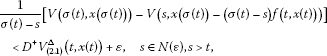where $x\left(t\right)$ is any solution of (2.1) and the upper right Dini derivative ${V}_{\ast }^{\mathrm{\Delta }}\left(t\right)$ of ${V}_{\ast }$ is given by

(2.12)

where ${V}_{\ast }\left(t\right)=V\left(t,x\left(t\right)\right)$.

Then it is well known that

${D}^{+}{V}_{\left(\text{2.1}\right)}^{\mathrm{\Delta }}\left(t,x\left(t\right)\right)={V}_{\ast }^{\mathrm{\Delta }}\left(t\right)$

if $V\left(t,x\right)$ is Lipschitzian in x for each $t\in \mathbb{T}$ [22, 40].

In case $t\in \mathbb{T}$ is right dense, we have

$\begin{array}{rcl}{D}^{+}{V}_{\left(\text{2.1}\right)}^{\mathrm{\Delta }}\left(t,x\left(t\right)\right)& =& D{V}_{\left(\text{2.1}\right)}\left(t,x\left(t\right)\right)=\underset{\eta \to 0+}{\overline{lim}}\frac{1}{\eta }\left[V\left(t+\eta ,x\left(t\right)+\eta f\left(t,x\left(t\right)\right)\right)-V\left(t,x\left(t\right)\right)\right]\\ =& \underset{\eta \to {0}^{+},\eta +t\in \mathbb{T}}{\overline{lim}}\frac{V\left(t+\eta ,x\left(t+\eta \right)\right)-V\left(t,x\left(t\right)\right)}{\eta }={D}^{+}{V}_{\ast }\left(t\right).\end{array}$

In case $t\in \mathbb{T}$ is right scattered and $V\left(t,x\left(t\right)\right)$ is continuous at t, we have

${D}^{+}{V}_{\left(\text{2.1}\right)}^{\mathrm{\Delta }}\left(t,x\left(t\right)\right)=\frac{1}{\mu \left(t\right)}\left[V\left(\sigma \left(t\right),x\left(\sigma \left(t\right)\right)\right)-V\left(t,x\left(t\right)\right)\right].$

In fact, if $x\left(t\right)$ is a solution of (2.1), we have

$\begin{array}{rcl}{V}^{\mathrm{\Delta }}\left(t,x\left(t\right)\right)& =& {V}^{{\mathrm{\Delta }}_{t}}\left(t,x\left(t\right)\right)+\left[{\int }_{0}^{1}{D}_{2}V\left(\sigma \left(t\right),x\left(t\right)+\eta \mu \left(t\right){x}^{\mathrm{\Delta }}\left(t\right)\right)\phantom{\rule{0.2em}{0ex}}d\eta \right]{x}^{\mathrm{\Delta }}\left(t\right)\\ =& {V}^{{\mathrm{\Delta }}_{t}}\left(t,x\left(\sigma \left(t\right)\right)\right)+\left[{\int }_{0}^{1}{D}_{2}V\left(t,x\left(t\right)+\eta \mu \left(t\right){x}^{\mathrm{\Delta }}\left(t\right)\right)\phantom{\rule{0.2em}{0ex}}d\eta \right]{x}^{\mathrm{\Delta }}\left(t\right),\end{array}$

by the chain rule of a differentiable function $V\left(t,x\left(t\right)\right)$ , Theorem 1].

We note that the total difference of the function V along the solutions x of (2.1) is given by

$\mathrm{\Delta }{V}_{\left(\text{2.1}\right)}\left(n,x\right)=V\left(n+1,x\left(n+1,n,x\right)\right)-V\left(n,x\left(n,n,x\right)\right).$

Choi et al.  investigated h-stability for nonlinear differential systems using the notions of ${t}_{\mathrm{\infty }}$-similarity and Lyapunov functions. Also, Choi et al.  introduced the notion of ${n}_{\mathrm{\infty }}$-summable similarity which is the corresponding ${t}_{\mathrm{\infty }}$-similarity for the discrete case and then characterized h-stability in variation and asymptotic equilibrium in variation for nonlinear difference system via ${n}_{\mathrm{\infty }}$-summable similarity and comparison principle.

Now, we define ${u}_{\mathrm{\infty }}$-similarity on time scales in order to unify (continuous ) ${t}_{\mathrm{\infty }}$-similarity and (discrete) ${n}_{\mathrm{\infty }}$-similarity for matrix-valued functions.

Let ${\mathfrak{M}}_{n}\left(\mathbb{R}\right)$ be the set of all $n×n$ invertible matrices over $\mathbb{R}$, and ${C}_{\mathrm{rd}}^{1}\left({\mathbb{T}}^{\kappa },{\mathfrak{M}}_{n}\left(\mathbb{R}\right)\right)$ be the set of all rd-continuous differentiable functions S from ${\mathbb{T}}^{\kappa }$ to ${\mathfrak{M}}_{n}\left(\mathbb{R}\right)$ such that S and ${S}^{-1}$ are bounded on ${\mathbb{T}}_{0}$.

Definition 2.3 A function $A:{\mathbb{T}}^{\kappa }\to {M}_{n}\left(\mathbb{R}\right)$ is called regressive if for each $t\in {\mathbb{T}}^{\kappa }$ the $n×n$ matrix $I+\mu \left(t\right)A\left(t\right)$ is invertible.

The class of all rd-continuous and regressive functions from ${\mathbb{T}}^{\kappa }$ to ${M}_{n}\left(\mathbb{R}\right)$ is denoted by

${C}_{\mathrm{rd}}\mathcal{R}\left({\mathbb{T}}^{\kappa },{M}_{n}\left(\mathbb{R}\right)\right).$

Definition 2.4 

Let $A,B\in {C}_{\mathrm{rd}}\mathcal{R}\left({\mathbb{T}}^{\kappa },{M}_{n}\left(\mathbb{R}\right)\right)$ and ${t}_{0}\in \mathbb{T}$. A function A is ${u}_{\mathrm{\infty }}$-similar to a function B if there exists an absolutely integrable function $F\in {C}_{\mathrm{rd}}\left(\mathbb{T},{M}_{n}\left(\mathbb{R}\right)\right)$, i.e., ${\int }_{{t}_{0}}^{\mathrm{\infty }}|F\left(t\right)|\mathrm{\Delta }t<\mathrm{\infty }$, such that

${S}^{\mathrm{\Delta }}\left(t\right)+{S}^{\sigma }\left(t\right)B\left(t\right)-A\left(t\right)S\left(t\right)=F\left(t\right),\phantom{\rule{1em}{0ex}}t\in {\mathbb{T}}^{\kappa },$
(2.13)

for some $S\in {C}_{\mathrm{rd}}^{1}\left({\mathbb{T}}^{\kappa },{\mathfrak{M}}_{n}\left(\mathbb{R}\right)\right)$.

Remark 2.5 If $\mathbb{T}=\mathbb{R}$, then ${u}_{\mathrm{\infty }}$-similarity becomes ${t}_{\mathrm{\infty }}$-similarity and if $\mathbb{T}=\mathbb{Z}$, then ${u}_{\mathrm{\infty }}$-similarity becomes ${n}_{\mathrm{\infty }}$-similarity. Also if A and B are ${u}_{\mathrm{\infty }}$-similar with $F=0$ defined on $\mathbb{T}$, then they are kinematically similar .

Let $\mathbb{N}\left({n}_{0}\right)=\left\{{n}_{0},{n}_{0}+1,\dots ,{n}_{0}+k,\dots \right\}$, where ${n}_{0}$ is a nonnegative integer and $\mathfrak{M}$ denote the set of all $s×s$ invertible matrix-valued functions defined on $\mathbb{N}\left({n}_{0}\right)$.

Remark 2.6 , Definition 2.5]

A matrix function $A\in \mathfrak{M}$ is ${n}_{\mathrm{\infty }}$-summably similar to a matrix function $B\in \mathfrak{M}$ if there exists an absolutely summable $s×s$ matrix $F\left(n\right)$ over $\mathbb{N}\left({n}_{0}\right)$, that is,

$\sum _{l={n}_{0}}^{\mathrm{\infty }}|F\left(l\right)|<\mathrm{\infty }$

such that

$\mathrm{\Delta }S\left(n\right)+S\left(n+1\right)B\left(n\right)-A\left(n\right)S\left(n\right)=F\left(n\right)$
(2.14)

for some $S\in \mathfrak{S}$.

For the example of ${n}_{\mathrm{\infty }}$-summable similarity, see .

Remark 2.7 We can easily show that the ${n}_{\mathrm{\infty }}$-summable similarity is an equivalence relation in the similar manner of Trench in . Also, if A and B are ${n}_{\mathrm{\infty }}$-summably similar with $F\left(n\right)=0$, then we say that they are kinematically similar.

Pinto  introduced the notion of h-stability which is an extension of the notions of exponential stability and uniform stability of the solutions of differential equations. The symbol $|\cdot |$ will be used to denote any convenient vector norm in ${\mathbb{R}}^{n}$. We recall the notions of h-stability for dynamic systems on time scales in .

Definition 2.8 System (2.1) is called an h-system if there exist a positive rd-continuous function $h:\mathbb{T}\to \mathbb{R}$, a constant $c\ge 1$ and $\delta >0$ such that

$|x\left(t,{t}_{0},{x}_{0}\right)|\le c|{x}_{0}|h\left(t\right)h{\left({t}_{0}\right)}^{-1},\phantom{\rule{1em}{0ex}}t\ge {t}_{0}$

for $|{x}_{0}|<\delta$ (here $h{\left(t\right)}^{-1}=\frac{1}{h\left(t\right)}$).

Moreover, system (2.1) is said to be

(h S) h-stable if h is a bounded function in the definition of h-system,

(Gh S) globally h-stable if system (2.1) is h S for every ${x}_{0}\in D$, where $D\subset {\mathbb{R}}^{n}$ is a region which includes the origin,

(h SV) h-stable in variation if system (2.3) is h S,

(Gh SV) globally h-stable in variation if system (2.3) is Gh S.

For the various definitions of stability, we refer to  and we obtain the following possible implications for system (2.1) among the various types of stability:

$\begin{array}{rcl}h\text{-stability}& ⇒& \text{uniform exponential stability}\\ ⇒& \text{uniform Lipschitz stability}\\ ⇒& \text{uniform stability}\end{array}$

as in [7, 34]. See [8, 26, 28, 29] for stability of nonlinear difference systems.

We consider two linear dynamic systems

${x}^{\mathrm{\Delta }}=A\left(t\right)x$
(2.15)

and

${y}^{\mathrm{\Delta }}=B\left(t\right)y,$
(2.16)

where $A,B\in {C}_{\mathrm{rd}}\mathcal{R}\left({\mathbb{T}}^{\kappa },{M}_{n}\left(\mathbb{R}\right)\right)$.

We say that if A and B are ${u}_{\mathrm{\infty }}$-similar, then systems (2.15) and (2.16) are ${u}_{\mathrm{\infty }}$-similar.

Lemma 2.5 , Lemma 2.3]

System (2.15) is an h-system if and only if there exist a positive rd-continuous function h defined on $\mathbb{T}$ and a constant $c\ge 1$ such that

$|{\mathrm{\Phi }}_{A}\left(t,{t}_{0}\right)|\le ch\left(t\right)h{\left({t}_{0}\right)}^{-1},\phantom{\rule{1em}{0ex}}t\in {\mathbb{T}}_{0},$

where ${\mathrm{\Phi }}_{A}$ is a fundamental matrix solution of (2.15).

We obtain the following result from Lemma 2.3 in .

Lemma 2.6 Assume that A and B are ${u}_{\mathrm{\infty }}$-similar. Then

${\mathrm{\Phi }}_{B}\left(t,{t}_{0}\right)={S}^{-1}\left(t\right)\left[{\mathrm{\Phi }}_{A}\left(t,{t}_{0}\right)S\left({t}_{0}\right)+{\int }_{{t}_{0}}^{t}{\mathrm{\Phi }}_{A}\left(t,\sigma \left(s\right)\right)F\left(s\right){\mathrm{\Phi }}_{B}\left(s,{t}_{0}\right)\mathrm{\Delta }s\right],\phantom{\rule{1em}{0ex}}t,{t}_{0}\in \mathbb{T},$

where ${\mathrm{\Phi }}_{A}$ and ${\mathrm{\Phi }}_{B}$ are the matrix exponential functions of (2.15) and (2.16) respectively.

Medina and Pinto , Theorem 3] showed that h SV implies h S. Also, they proved the converse when the condition

$\sum _{l={n}_{0}}^{\mathrm{\infty }}\frac{h\left(l\right)}{h\left(l+1\right)}|{f}_{x}\left(l,{n}_{0},{x}_{0}\right)-{f}_{x}\left(l,0\right)|<\mathrm{\infty },\phantom{\rule{1em}{0ex}}{n}_{0}\ge 0$
(2.17)

for $|{x}_{0}|\le \delta$ holds , Theorem 14].

In order to establish our main results, we will introduce the following condition.

(H): ${f}_{x}\left(t,0\right)$ and ${f}_{x}\left(t,x\left(t,{t}_{0},{x}_{0}\right)\right)$ are ${u}_{\mathrm{\infty }}$-similar for $t\ge {t}_{0}$ and $|{x}_{0}|\le \delta$ for some constant $\delta >0$ and ${\int }_{{t}_{0}}^{\mathrm{\infty }}\frac{h\left(t\right)}{h\left(\sigma \left(t\right)\right)}|F\left(t\right)|\mathrm{\Delta }t<\mathrm{\infty }$ with the positive rd-continuous function $h\left(t\right)$ defined on $\mathbb{T}$.

Lemma 2.7 , Theorem 3.4]

Assume that condition (H) is satisfied. Then variational system (2.2) is also an h-system if and only if variational system (2.3) is an h-system.

We can obtain the same result about Lemma 2.7 by assuming that ${f}_{x}\left(t,0\right)$ and ${f}_{x}\left(t,x\left(t,{t}_{0},{x}_{0}\right)\right)$ are ${u}_{\mathrm{\infty }}$-quasisimilar for $t\ge {t}_{0}$ instead of the condition (H) in Lemma 2.7 , Theorem 3.3].

For nonlinear dynamic system (2.1), we can show that

$GhSV⇔GhS,\phantom{\rule{2em}{0ex}}hS⇔hSV$

by using the concept of ${u}_{\mathrm{\infty }}$-similarity.

We study the relation of h-stability between two systems (2.1) and (2.3) by assuming the condition (H) is satisfied.

Theorem 2.8 , Theorem 2]

Suppose that condition (H) is satisfied. If $x=0$ of (2.1) is h-stable, then $v=0$ of (2.2) is h-stable.

We obtain the following result from (2.8).

Theorem 2.9 If $z=0$ of (2.3) is h-stable, then $x=0$ of (2.1) is h-stable.

We can obtain the following result by using Lemma 2.7 and Theorem 2.8.

Theorem 2.10 Assume that condition (H) is satisfied. If $x=0$ of (2.1) is h-stable, then $z=0$ of (2.1) is h-stable in variation.

Remark 2.9 For nonlinear dynamic system (2.1), we show that two concepts of h-stability and h-stability in variation are equivalent under the condition that two variational systems (2.2) and (2.3) are ${u}_{\mathrm{\infty }}$-similar.

Choi et al. investigated Massera type converse theorems for the nonlinear difference system $x\left(n+1\right)=f\left(n,x\left(n\right)\right)$ via ${n}_{\mathrm{\infty }}$-similarity in , Theorem 5] and , Theorem 2.1]. Furthermore, they characterized h-stability in variation for the nonlinear difference system by using the notion of ${n}_{\mathrm{\infty }}$-summable similarity in .

We need the following lemma to prove our main theorem.

Lemma 2.11 , Korollar 2.1.13]

If the delta differentiable function $h:\mathbb{T}\to \mathbb{R}$ is positive, then $\frac{{h}^{\mathrm{\Delta }}\left(t\right)}{h\left(t\right)}$ is positively regressive, and ${e}_{p}\left(t,{t}_{0}\right)$ satisfies

${e}_{p}\left(t,{t}_{0}\right)=\frac{h\left(t\right)}{h\left({t}_{0}\right)},$

where $p\left(t\right)=\frac{{h}^{\mathrm{\Delta }}\left(t\right)}{h\left(t\right)}$.

We can obtain the following result that characterizes h-stability for nonlinear dynamic system (2.1) via the notions of Lyapunov functions and ${u}_{\mathrm{\infty }}$-similarity. It is adapted from Theorem 3.6.1 in  and Theorem 3.1 in .

Theorem 2.12 Assume that condition (H) is satisfied. Suppose further that ${h}^{\mathrm{\Delta }}\left(t\right)$ exists and is rd-continuous on $\mathbb{T}$. Then system (2.1) is GhS if and only if there exists a function $V\left(t,x\right)$ defined on $\mathbb{T}×{\mathbb{R}}^{n}$ such that the following properties hold:

1. (i)

$|x|\le V\left(t,x\right)|\le c|x|$ for $\left(t,x\right)\in \mathbb{T}×{\mathbb{R}}^{n}$ and a constant $c\ge 1$;

2. (ii)

$|V\left(t,{x}_{1}\right)-V\left(t,{x}_{2}\right)|\le c|{x}_{1}-{x}_{2}|$ for $t\in \mathbb{T}$ and ${x}_{1},{x}_{2}\in {\mathbb{R}}^{n}$;

3. (iii)

${V}^{\mathrm{\Delta }}\left(t,x\right)\le \frac{{h}^{\mathrm{\Delta }}\left(t\right)}{h\left(t\right)}V\left(t,x\right)$ for $\left(t,x\right)\in \mathbb{T}×{\mathbb{R}}^{n}$;

4. (iv)

$V\left(t,x\right)$ is continuous on $\mathbb{T}×{\mathbb{R}}^{n}$;

$\underset{\left(\stackrel{ˆ}{t},\stackrel{ˆ}{x}\right)\to \left(t,x\right),\stackrel{ˆ}{t}\ge t}{lim}|V\left(\stackrel{ˆ}{t},\stackrel{ˆ}{x}\right)-V\left(t,x\right)|=0.$

Proof Necessity: Suppose that system (2.1) is Gh S. Then system (2.1) is Gh SV by Theorem 2.10, i.e., there exist a constant $c\ge 1$ and a positive rd-continuous bounded function h defined on $\mathbb{T}$ such that for each $x\in {\mathbb{R}}^{n}$

$|\mathrm{\Phi }\left(t,{t}_{0},{x}_{0}\right)|\le ch\left(t\right)h{\left({t}_{0}\right)}^{-1},\phantom{\rule{1em}{0ex}}t\ge {t}_{0},$
(2.18)

where Φ is a fundamental matrix solution of (2.3).

Fix $t\in \mathbb{T}$. Let ${A}_{t}:=\left\{\tau \in {\mathbb{R}}_{+}:t+\tau \in \mathbb{T}\right\}$. Then we note that ${A}_{t}$ is nonempty from $0\in {A}_{t}$.

Define the function $V:\mathbb{T}×{\mathbb{R}}^{n}\to {\mathbb{R}}_{+}$ by

$V\left(t,x\right)=\underset{\tau \in {\mathbb{A}}_{t}}{sup}|x\left(t+\tau ,t,x\right)|h{\left(t+\tau \right)}^{-1}h\left(t\right),$

where $x\left(t+\tau ,t,x\right)$ is a unique solution of system (2.1) for $\left(t,x\right)\in \mathbb{T}×{\mathbb{R}}^{n}$ with the initial value $x\left(t,t,x\right)=x$. From Gh S of (2.1) we have

$|x\left(t,{t}_{0},{x}_{0}\right)|\le c|{x}_{0}|h\left(t\right)h{\left({t}_{0}\right)}^{-1},\phantom{\rule{1em}{0ex}}t\in {\mathbb{T}}_{0},|{x}_{0}|<\mathrm{\infty }.$

Furthermore, we obtain

$\begin{array}{rcl}|x|=|x\left(t,t,x\right)|& \le & \underset{\tau \in {\mathbb{A}}_{t}}{sup}|x\left(t+\tau ,t,x\right)|h{\left(t+\tau \right)}^{-1}h\left(t\right)\\ \le & c|x|h\left(t+\tau \right)h{\left(t\right)}^{-1}h{\left(t+\tau \right)}^{-1}h\left(t\right)=c|x|.\end{array}$

Thus V satisfies property (i).

Let $\left(t,{x}_{1}\right),\left(t,{x}_{2}\right)\in \mathbb{T}×{\mathbb{R}}^{n}$. Then we have

$\begin{array}{rcl}|V\left(t,{x}_{1}\right)-V\left(t,{x}_{2}\right)|& =& |\underset{\tau \in {\mathbb{A}}_{t}}{sup}|x\left(t+\tau ,t,{x}_{1}\right)|h{\left(t+\tau \right)}^{-1}h\left(t\right)\\ -\underset{\tau \in {\mathbb{A}}_{t}}{sup}|x\left(t+\tau ,t,{x}_{2}\right)|h{\left(t+\tau \right)}^{-1}h\left(t\right)|\\ \le & \underset{\tau \in {\mathbb{A}}_{t}}{sup}|\left(x\left(t+\tau ,t,{x}_{1}\right)-x\left(t+\tau ,t,{x}_{2}\right)\right)|h{\left(t+\tau \right)}^{-1}h\left(t\right).\end{array}$
(2.19)

It follows from Lemma 2.2 that for each ${x}_{1}$ and ${x}_{2}$ in a convex subset D of ${\mathbb{R}}^{n}$

$|x\left(t,{t}_{0},{x}_{1}\right)-x\left(t,{t}_{0},{x}_{2}\right)|\le |{x}_{1}-{x}_{2}|\underset{\eta \in D}{sup}|\mathrm{\Phi }\left(t,{t}_{0},\eta \right)|.$
(2.20)

In view of (2.18), (2.19) and (2.20), we have

$\begin{array}{rcl}|V\left(t,{x}_{1}\right)-V\left(t,{x}_{2}\right)|& \le & |{x}_{1}-{x}_{2}|\underset{\tau \in {\mathbb{A}}_{t},\eta \in D}{sup}|\mathrm{\Phi }\left(t+\tau ,t,\eta \right)|h{\left(t+\tau \right)}^{-1}h\left(t\right)\\ \le & |{x}_{1}-{x}_{2}|c|h\left(t+\tau \right)h{\left(t\right)}^{-1}h{\left(t+\tau \right)}^{-1}h\left(t\right)\\ \le & c|{x}_{1}-{x}_{2}|,\phantom{\rule{1em}{0ex}}t\in {\mathbb{T}}_{0},{x}_{1},{x}_{2}\in {\mathbb{R}}^{n}.\end{array}$

This implies that $V\left(t,x\right)$ is globally Lipschitzian in x for fixed $t\in \mathbb{T}$.

Next, we will prove property (iii). Let $x\left(t,{t}_{0},{x}_{0}\right)$ be a unique solution of system (2.1) for each initial point $\left({t}_{0},{x}_{0}\right)\in \mathbb{T}×{\mathbb{R}}^{n}$. We will consider two cases, $\sigma \left(t\right)=t$ and $\sigma \left(t\right)>t$, in the proof.

Suppose that $\sigma \left(t\right)=t$ and let $\delta \in {A}_{t}$. By the uniqueness of solutions of (2.1) and the definition of h S, we have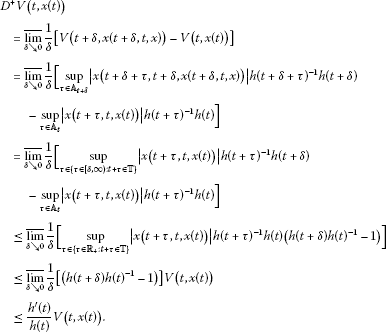Suppose that $\sigma \left(t\right)>t$. Then it follows from the definition of ${V}^{\mathrm{\Delta }}\left(t\right)$ that

${V}_{\ast }^{\mathrm{\Delta }}\left(t\right)=\frac{V\left(\sigma \left(t\right)\right)-V\left(t\right)}{\mu \left(t\right)}.$

Since the solution of (2.1) is unique, we have the following derivative:

$\begin{array}{rcl}{V}_{\ast }^{\mathrm{\Delta }}\left(t\right)& =& \frac{V\left(\sigma \left(t\right)\right)-V\left(t\right)}{\mu \left(t\right)}\\ =& \frac{1}{\mu \left(t\right)}\left[\underset{\tau \in {\mathbb{A}}_{\sigma \left(t\right)}}{sup}|x\left(\sigma \left(t\right)+\tau ,\sigma \left(t\right),x\left(\sigma \left(t\right),t,x\right)\right)|h{\left(\sigma \left(t\right)+\tau \right)}^{-1}h\left(\sigma \left(t\right)\right)\\ -\underset{\tau \in {\mathbb{A}}_{t}}{sup}|x\left(t+\tau ,t,x\left(t\right)\right)|h{\left(t+\tau \right)}^{-1}h\left(t\right)\right]\\ =& \frac{1}{\mu \left(t\right)}\left[\underset{\tau \in {\mathbb{A}}_{t+\mu \left(t\right)}}{sup}|x\left(t+\mu \left(t\right)+\tau ,t+\mu \left(t\right),\\ x\left(t+\mu \left(t\right),t,x\right)\right)|h{\left(t+\mu \left(t\right)+\tau \right)}^{-1}h\left(t+\mu \left(t\right)\right)\\ -\underset{\tau \in {\mathbb{A}}_{t}}{sup}|x\left(t+\tau ,t,x\left(t\right)\right)|h{\left(t+\tau \right)}^{-1}h\left(t\right)\right]\\ =& \frac{1}{\mu \left(t\right)}\left[\underset{\tau \in \left\{\tau \in \left[\mu \left(t\right),\mathrm{\infty }\right):t+\tau \in \mathbb{T}\right\}}{sup}|x\left(t+\tau ,t,x\left(t\right)\right)|h{\left(t+\tau \right)}^{-1}h\left(t+\mu \left(t\right)\right)\\ -\underset{\tau \in {\mathbb{A}}_{t}}{sup}|x\left(t+\tau ,t,x\left(t\right)\right)|h{\left(t+\tau \right)}^{-1}h\left(t\right)\right]\\ \le & \frac{1}{\mu \left(t\right)}\left[h\left(t+\mu \left(t\right)\right)h{\left(t\right)}^{-1}-1\right]V\left(t,x\left(t\right)\right)\\ =& \frac{{h}^{\mathrm{\Delta }}\left(t\right)}{h\left(t\right)}V\left(t,x\left(t\right)\right).\end{array}$

Thus property (iii) was satisfied for two cases.

The continuity of $V\left(t,x\right)$ can be proved in a similar manner of Theorem 3.6.1 in  and Theorem 3.1 in . It remains to show that V is continuous in the sense of (iv): let $t\in \mathbb{T}$, $x\in {\mathbb{R}}^{n}$ be fixed and choose $\epsilon >0$ arbitrary. Then ${\delta }_{1}>0$ and ${\delta }_{2}>0$ must be found such that

$|V\left(\stackrel{ˆ}{t},\stackrel{ˆ}{x}\right)-V\left(t,x\right)|<\epsilon$

holds for all

$\stackrel{ˆ}{t}=t+\nu ,\phantom{\rule{1em}{0ex}}\nu \in {A}_{t},0\le \nu <{\delta }_{1}$
(2.21)

and all $\stackrel{ˆ}{x}\in {B}_{{\delta }_{2}}\left(x\right)$, where ${B}_{{\delta }_{2}}\left(x\right)$ is an open ball centered on x of radius ${\delta }_{2}$.

If $t\in \mathbb{T}$ is right scattered, then we can always choose a suitable ${\delta }_{1}$ such that $\stackrel{ˆ}{t}=t$ is the only point satisfying condition (2.21) (see , Theorem 3.6.1]). Thus $V\left(t,x\right)$ is continuous in $\left(t,x\right)\in \mathbb{T}×{\mathbb{R}}^{n}$ since V is globally Lipschitz continuous in x for fixed $t\in \mathbb{T}$.

Suppose that $t\in \mathbb{T}$ is right dense and let $\stackrel{ˆ}{t}=t+\nu$ for $\nu \in {A}_{t}$ with $\nu \ge 0$. Then we have

$\begin{array}{rcl}|V\left(\stackrel{ˆ}{t},\stackrel{ˆ}{x}\right)-V\left(t,x\right)|& =& |V\left(t+\nu ,\stackrel{ˆ}{x}\right)-V\left(t,x\right)|\\ \le & |V\left(t+\nu ,\stackrel{ˆ}{x}\right)-V\left(t+\nu ,x\right)|\end{array}$
(2.22)
$+|V\left(t+\nu ,x\right)-V\left(t+\nu ,x\left(t+\nu ,t,x\right)\right)|$
(2.23)
$+|V\left(t+\nu ,x\left(t+\nu ,t,x\right)\right)-V\left(t,x\right)|.$
(2.24)

Since $V\left(t,x\right)$ is Lipschitzian in x and $x\left(t+\nu ,t,x\right)$ is continuous in ν, the first two terms (2.22) and (2.23) on the right-hand side of the preceding inequality are small when $|\stackrel{ˆ}{x}-x|$ and ν are small. That is, we have

$|V\left(t+\nu ,\stackrel{ˆ}{x}\right)-V\left(t+\nu ,x\right)|<\frac{\epsilon }{3}$

for all $\stackrel{ˆ}{x}\in {B}_{{\delta }_{2}}\left(x\right)$ when ${\delta }_{2}<\frac{\epsilon }{3c}$ and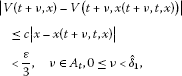since it follows from ${lim}_{\nu \to 0,\nu \in {A}_{t}}x\left(t+\nu ,t,x\right)=x$ that there exists a ${\stackrel{ˆ}{\delta }}_{1}>0$ such that

$|x-x\left(t+\nu ,t,x\right)|<\frac{\epsilon }{3c}$

for all $\nu \in {A}_{t}$ with $0\le \nu <{\stackrel{ˆ}{\delta }}_{1}$.

Let us consider the third term in (2.24). We note that

$x\left(t+\nu +\tau ,t+\nu ,x\left(t+\nu ,t,x\right)\right)=x\left(t+\nu +\tau ,t,x\right).$

Thus we have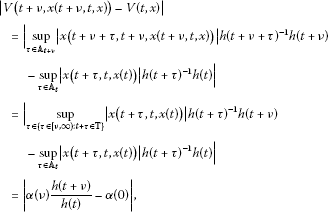where $\alpha \left(\nu \right)={sup}_{\tau \in \left\{\tau \in \left[\nu ,\mathrm{\infty }\right):t+\tau \in \mathbb{T}\right\}}|x\left(t+\tau ,t,x\left(t\right)\right)|h{\left(t+\tau \right)}^{-1}h\left(t\right)$ for $\nu \in {A}_{t}$.

We have $\alpha \left(\nu \right)\le \alpha \left(0\right)$ for all $\nu \in {A}_{t}$ with $\nu \ge 0$. Furthermore, $\alpha \left(\nu \right)$ is a nonincreasing function in ν with

$\underset{\nu \to 0,\nu \in {A}_{t}}{lim}\alpha \left(\nu \right)=\alpha \left(0\right).$

Hence, there exists a ${\stackrel{ˆ}{\delta }}_{3}>0$ such that

$|\alpha \left(\nu \right)\frac{h\left(t+\nu \right)}{h\left(t\right)}-\alpha \left(0\right)|<\frac{\epsilon }{3},\phantom{\rule{1em}{0ex}}\nu \in {A}_{t},0\le \nu \le {\stackrel{ˆ}{\delta }}_{3}.$

Now, choose ${\delta }_{1}=min\left\{{\stackrel{ˆ}{\delta }}_{1},{\stackrel{ˆ}{\delta }}_{3}\right\}$. For $\stackrel{ˆ}{t}=t+\nu$ with $\nu \in {A}_{t}$ where $0\le \nu <{\delta }_{1}$ and $\stackrel{ˆ}{x}\in {B}_{{\delta }_{2}}\left(x\right)$, combining all of the above estimates of the terms in (2.22)-(2.24) gives

$|V\left(\stackrel{ˆ}{t},\stackrel{ˆ}{x}\right)-V\left(t,x\right)|<\frac{\epsilon }{3}+\frac{\epsilon }{3}+\frac{\epsilon }{3}=\epsilon ,$

which proves the continuity of $V\left(t,x\right)$.

Sufficiency: Assume that $V\left(t,x\right)$ satisfies the properties (i)-(iv). Let $x\left(t,{t}_{0},{x}_{0}\right)$ be any solution of system (2.1). Then it follows from condition (iii) of $V\left(t,x\right)$ that

$V\left(t,x\left(t\right)\right)\le V\left({t}_{0},{x}_{0}\right)+{\int }_{{t}_{0}}^{t}\frac{{h}^{\mathrm{\Delta }}\left(s\right)}{h\left(s\right)}V\left(s,x\left(s\right)\right)\mathrm{\Delta }s,\phantom{\rule{1em}{0ex}}t\ge {t}_{0}.$

From Gronwall’s inequality on time scale  and Lemma 2.15 , we obtain

$\begin{array}{rcl}V\left(t,x\left(t\right)\right)& \le & V\left({t}_{0},{x}_{0}\right){e}_{p\left(t\right)}\left(t,{t}_{0}\right)\\ \le & V\left({t}_{0},{x}_{0}\right)h\left(t\right)h{\left({t}_{0}\right)}^{-1},\phantom{\rule{1em}{0ex}}t\ge {t}_{0},\end{array}$
(2.25)

where $p\left(t\right)=\frac{{h}^{\mathrm{\Delta }}\left(t\right)}{h\left(t\right)}$. From (2.25) and property (i) of $V\left(t,x\right)$, we have

$|x\left(t,{t}_{0},{x}_{0}\right)|\le c|{x}_{0}|h\left(t\right)h{\left({t}_{0}\right)}^{-1},$

for each $t\ge {t}_{0}$ and ${x}_{0}\in {\mathbb{R}}^{n}$. Hence the zero solution $x=0$ of (2.1) is Gh S. This completes the proof of the theorem. □

Remark 2.10 Assume that condition (H) is satisfied for $\mathbb{T}=\mathbb{R}$. Furthermore, suppose that ${h}^{\prime }\left(t\right)$ exists and is continuous on ${\mathbb{R}}_{+}$. Then we can obtain Theorems 2.4 and 2.6 in  as a continuous version of Theorem 2.12.

Also, we can obtain the following result as a discrete version of Theorem 2.12.

Corollary 2.13 , Theorem 3.7]

Assume that ${f}_{x}\left(n,0\right)$ is ${n}_{\mathrm{\infty }}$-summably similar to ${f}_{x}\left(n,x\left(n,{n}_{0},{x}_{0}\right)\right)$ for $n\ge {n}_{0}\ge 0$ and every ${x}_{0}\in {\mathbb{R}}^{m}$ with $\frac{h\left(n\right)}{h\left(n+1\right)}|F\left(n\right)|\in {l}_{1}\left(\mathbb{N}\left({n}_{0}\right)\right)$. Then system (2.1) is GhS if and only if there exists a function $V\left(n,z\right)$ defined on $\mathbb{N}\left({n}_{0}\right)×{\mathbb{R}}^{m}$ such that the following properties hold:

1. (i)

$V\left(n,z\right)$ is continuous on $\mathbb{N}\left({n}_{0}\right)×{\mathbb{R}}^{m}$;

2. (ii)

$|x-y|\le V\left(n,x-y\right)|\le c|x-y|$ for $\left(n,x,y\right)\in \mathbb{N}\left({n}_{0}\right)×{\mathbb{R}}^{m}×{\mathbb{R}}^{m}$;

3. (iii)

$|V\left(n,{z}_{1}\right)-V\left(n,{z}_{2}\right)|\le c|{z}_{1}-{z}_{2}|$ for $n\in \mathbb{N}\left({n}_{0}\right)$, ${z}_{1},{z}_{2}\in {\mathbb{R}}^{m}$;

4. (iv)

$\frac{\mathrm{\Delta }V\left(n,x-y\right)}{V\left(n,x-y\right)}\le \frac{\mathrm{\Delta }h\left(n\right)}{h\left(n\right)}$ for $\left(n,x,y\right)\in \mathbb{N}\left({n}_{0}\right)×{\mathbb{R}}^{m}×{\mathbb{R}}^{m}$ with $x\ne y$.

Remark 2.11 Choi et al. [8, 9] introduced the notion of ${n}_{\mathrm{\infty }}$-similarity which is slightly different from ${n}_{\mathrm{\infty }}$-summable similarity and studied a general variational stability for a nonlinear difference system via ${n}_{\mathrm{\infty }}$-similarity and Lyapunov functions. We can obtain the discrete analogues , Theorem 5, Corollary 8] and , Theorem 2.1] as a discrete version of Theorem 2.12.

Remark 2.12 Choi et al. , Theorem 2.16] studied h-stability for linear dynamic equations on time scales by using the unified time scale quadratic Lyapunov functions. Also, Mukdasai and Niamsup , Theorem 3.13] derived a sufficient condition for h-stability for a linear time-varying system with nonlinear perturbation on time scales by constructing appropriate Lyapunov functions.

We can obtain the following Massera type converse theorem for the uniform exponential asymptotic stability of linear dynamic equations on time scales as a special case of Theorem 2.12.

Corollary 2.14 , Theorem 3.1]

Assume that $f\left(t,x\right)=A\left(t\right)x$ is linear, where $A\in {C}_{\mathrm{rd}}\mathcal{R}\left(\mathbb{T},{\mathbb{R}}^{n}×{\mathbb{R}}^{n}\right)$. If system (2.1) is hS with $h\left(t\right)={e}^{-\lambda t}$ on time scales $\mathbb{T}$ for a nonnegative constant λ, then there exists a function $V:\mathbb{T}×{\mathbb{R}}^{n}\to {\mathbb{R}}^{n}$ such that

1. (i)

$|x|\le V\left(t,x\right)\le K|x|$ for all $t\in \mathbb{T},x\in {\mathbb{R}}^{n}$.

2. (ii)

$|V\left(t,{x}_{1}\right)-V\left(t,{x}_{2}\right)|\le K|{x}_{1}-{x}_{2}|$ for any fixed $t\in \mathbb{T}$ and all ${x}_{1},{x}_{2}\in {\mathbb{R}}^{n}$.

3. (iii)

The upper right Dini derivative of ${V}_{\ast }$ exists and the estimates ${V}_{\ast }^{\mathrm{\Delta }}\left(t\right)\le {\overline{\xi }}_{-\lambda }\left(t\right){V}_{\ast }\left(t\right)<0$ hold for all $t\ge {t}_{0}$ for a positive constants K and λ. Here the function ${\overline{\xi }}_{\lambda }:\mathbb{T}\to \mathbb{R}$ is given by

${\overline{\xi }}_{\lambda }\left(t\right)=\left\{\begin{array}{cc}\frac{exp\left(\mu \left(t\right)\lambda \right)-1}{\mu \left(t\right)},\hfill & t<\sigma \left(t\right),\hfill \\ \lambda ,\hfill & t=\sigma \left(t\right).\hfill \end{array}$
4. (iv)

V is continuous from the right in $\left(t,x\right)\in \mathbb{T}×{\mathbb{R}}^{n}$, that is,

$\underset{\left(\stackrel{ˆ}{t},\stackrel{ˆ}{x}\right)\to \left(t,x\right),\stackrel{ˆ}{t}\ge t}{lim}|V\left(\stackrel{ˆ}{t},\stackrel{ˆ}{x}\right)-V\left(t,x\right)|=0.$

## 3 Examples

In this section we give some examples which illustrate some results from the previous section.

To illustrate the notion of ${u}_{\mathrm{\infty }}$-similarity, we will give an example for scalar functions defined on time scales.

Example 3.1 Let $a,b:\mathbb{T}\to \mathbb{R}$ be scalar functions given by

$a\left(t\right)={e}_{\alpha }\left(t,{t}_{0}\right)-1,\phantom{\rule{2em}{0ex}}b\left(t\right)=\frac{1}{2}{e}_{\alpha }\left(t,{t}_{0}\right)-1,$

where α is a negative regressive constant and ${lim}_{t\to \mathrm{\infty }}{e}_{\alpha }\left(t,{t}_{0}\right)=0$ for fixed ${t}_{0}\in \mathbb{T}$. If we put $s\left(t\right)=1$ for each $t\in \mathbb{T}$, then $s\left(t\right)$ and ${s}^{-1}\left(t\right)$ are bounded and nonzero functions. Moreover, we have

${s}^{\mathrm{\Delta }}\left(t\right)+s\left(\sigma \left(t\right)\right)b\left(t\right)-a\left(t\right)s\left(t\right)=-\frac{1}{2}{e}_{\alpha }\left(t,{t}_{0}\right)=f\left(t\right),\phantom{\rule{1em}{0ex}}t\in {\mathbb{T}}^{\kappa },$

where $f\left(t\right)=-\frac{1}{2}{e}_{\alpha }\left(t,{t}_{0}\right)$.

Thus we have

${\int }_{{t}_{0}}^{\mathrm{\infty }}|f\left(t\right)|\mathrm{\Delta }t={\int }_{{t}_{0}}^{\mathrm{\infty }}\frac{1}{2}{e}_{\alpha }\left(t,{t}_{0}\right)\mathrm{\Delta }t\le -\frac{1}{2\alpha }<\mathrm{\infty }.$

This implies that a and b are ${u}_{\mathrm{\infty }}$-similar on $\mathbb{T}$.

For the examples of nonscalar functions about ${t}_{\mathrm{\infty }}$-similarity on $\mathbb{T}=\mathbb{R}$ and ${n}_{\mathrm{\infty }}$-summable similarity on $\mathbb{T}=\mathbb{Z}$, see , Example] and , Example 2.6] respectively.

Example 3.2 To illustrate Lemma 2.5, we consider the linear dynamic system

${x}^{\mathrm{\Delta }}=A\left(t\right)x=\left(\begin{array}{cc}\frac{-{e}^{-t}}{2+{e}^{-t}}& 0\\ 0& 0\end{array}\right)x,\phantom{\rule{1em}{0ex}}x\left({t}_{0}\right)={x}_{0},t\ge {t}_{0}\in \mathbb{T},$
(3.1)

where $A\left(t\right)=\left(\begin{array}{cc}\frac{-{e}^{-t}}{2+{e}^{-t}}& 0\\ 0& 0\end{array}\right)$. If $\mu \left(t\right)<2{e}^{t}+1$ for $t\in \mathbb{T}$, then (3.1) is h-stable.

Proof A matrix exponential function ${\mathrm{\Phi }}_{A}\left(t,{t}_{0}\right)$ of (3.1) is given by

${\mathrm{\Phi }}_{A}\left(t,{t}_{0}\right)=\left(\begin{array}{cc}{e}_{p}\left(t,{t}_{0}\right)& 0\\ 0& 1\end{array}\right),$

where $p\left(t\right)=\frac{-{e}^{-t}}{2+{e}^{-t}}$ and ${e}_{p}\left(t,{t}_{0}\right)=exp{\int }_{{t}_{0}}^{t}{\xi }_{\mu \left(\tau \right)}\left(p\left(\tau \right)\right)\mathrm{\Delta }\tau$. Here the cylinder transformation ${\xi }_{\mu }\left(z\right)$ is given by

It follows that

for each $t,{t}_{0}\in \mathbb{T}$ with $t\ge {t}_{0}$, where M is a positive constant. Thus we obtain

$|{\mathrm{\Phi }}_{A}\left(t,{t}_{0}\right)|=|\left(\begin{array}{cc}{e}_{p}\left(t,{t}_{0}\right)& 0\\ 0& 1\end{array}\right)|\le ch\left(t\right)h{\left({t}_{0}\right)}^{-1},\phantom{\rule{1em}{0ex}}t\ge {t}_{0},$

where $h\left(t\right)={e}_{p}\left(t,{t}_{1}\right)$ is a positive bounded rd-continuous function for a fixed point ${t}_{1}\in \mathbb{T}$, and $c:=M+1$ is a positive constant. Hence system (3.1) is h-stable by Lemma 2.5. □

To illustrate that the converse of Theorem 2.8 does not hold in general, we give the following example.

Example 3.3 , Example 5.2]

Let $\mathbb{T}$ be the unbounded above time scales with $\mu \left(t\right)<2$ for each $t\in \mathbb{T}$. We consider the nonlinear dynamic equation

${x}^{\mathrm{\Delta }}=f\left(t,x\right)=-\frac{1}{2}x+{x}^{2},\phantom{\rule{1em}{0ex}}x\left({t}_{0}\right)={x}_{0}=1$
(3.2)

and its variational dynamic equation

${v}^{\mathrm{\Delta }}\left(t\right)={f}_{x}\left(t,0\right)v\left(t\right)=-\frac{1}{2}v\left(t\right),\phantom{\rule{1em}{0ex}}v\left({t}_{0}\right)={v}_{0}\ne 0,$
(3.3)

where ${f}_{x}\left(t,x\right)=-\frac{1}{2}+2x$. Then $v=0$ of (3.3) is h-stable, but $x=0$ of (3.2) is not h-stable.

Proof Since the fundamental solution is $\varphi \left(t\right)={e}_{-\frac{1}{2}}\left(t,{t}_{0}\right){v}_{0}$ for each $t\ge {t}_{0}$, Eq. (3.3) is h-stable with a positive bounded function $h\left(t\right)={e}_{-\frac{1}{2}}\left(t,{\stackrel{ˆ}{t}}_{0}\right)$ for a fixed point ${\stackrel{ˆ}{t}}_{0}\in \mathbb{T}$. But (3.2) is not h-stable because there exists an unbounded solution $x\left(t,{\stackrel{ˆ}{t}}_{0},1\right)$ of (3.2) such that

$x\left(t,{\stackrel{ˆ}{t}}_{0},1\right)=x\left(t\right)>t,\phantom{\rule{1em}{0ex}}t\in {\mathbb{T}}_{0}.$

□

## References

1. Agarwal RP: Difference Equations and Inequalities. 2nd edition. Dekker, New York; 2000.

2. Aulbach B, Hilger S: A unified approach to continuous and discrete dynamics. Colloq. Math. Soc. János Bolyai 53. In Qualitative Theory of Differential Equations (Szeged, 1988). North Holland, Amsterdam; 1990:37–56.

3. Aulbach B, Hilger S: Linear dynamic processes with inhomogeneous time scale. Math. Res. 59. In Nonlinear Dynamics and Quantum Dynamical Systems (Gaussig, 1990). Akademie, Berlin; 1990:9–20.

4. Aulbach B, Pötzsche C: Reducibility of linear dynamic equations on measure chains. J. Comput. Appl. Math. 2002, 141: 101–115. 10.1016/S0377-0427(01)00438-1

5. Bohner M, Peterson A: Dynamic Equations on Time Scales, An Introduction with Applications. Birkhäuser, Boston; 2001.

6. Bohner M, Peterson A: Advances in Dynamic Equations on Time Scales. Birkhäuser, Boston; 2003.

7. Choi SK, Koo NJ, Ryu HS: h -stability of differential systems via ${t}_{\mathrm{\infty }}$-similarity. Bull. Korean Math. Soc. 1997, 34: 371–383.

8. Choi SK, Koo NJ:Variationally stable difference systems by ${n}_{\mathrm{\infty }}$-similarity. J. Math. Anal. Appl. 2000, 249: 553–568. 10.1006/jmaa.2000.6910

9. Choi SK, Koo NJ, Goo YH: Variationally stable difference systems. J. Math. Anal. Appl. 2001, 256: 587–605. 10.1006/jmaa.2000.7330

10. Choi SK, Koo NJ, Song SM: h -stability for nonlinear perturbed difference systems. Bull. Korean Math. Soc. 2004, 41: 435–450.

11. Choi SK, Goo YH, Koo NJ: Variationally asymptotically stable difference systems. Adv. Differ. Equ. 2007., 2007: Article ID 35378

12. Choi SK, Kang W, Koo N: On h -stability of linear dynamic equations on time scales via ${u}_{\mathrm{\infty }}$ -similarity. J. Chungcheong Math. Soc. 2008, 21: 395–401.

13. Choi SK, Koo NJ, Dontha S: Asymptotic property in variation for nonlinear differential systems. Appl. Math. Lett. 2005, 18: 117–126. 10.1016/j.aml.2003.07.019

14. Choi SK, Koo NJ, Im DM: h -stability for linear dynamic equations on time scales. J. Math. Anal. Appl. 2006, 324: 707–720. 10.1016/j.jmaa.2005.12.046

15. Choi SK, Im DM, Koo N: Stability of linear dynamic systems on time scales. Adv. Differ. Equ. 2008., 2008: Article ID 670203

16. Choi SK, Goo YH, Koo N: h -stability of dynamic equations on time scales with nonregressivity. Abstr. Appl. Anal. 2008., 2008: Article ID 632473

17. Choi SK, Koo N: On the stability of linear dynamic systems on time scales. J. Differ. Equ. Appl. 2009, 15: 167–183. 10.1080/10236190802008528

18. Choi SK, Koo N: Stability of linear dynamic equations on time scales. Discrete Contin. Dyn. Syst. 2009, 2009: 161–170. 7th AIMS Conference, suppl.

19. Conti R:Sulla ${t}_{\mathrm{\infty }}$-similitudine tra matrici e la stabilità dei sistemi differenziali lineari. Atti Accad. Naz. Lincei, Rend. Cl. Sci. Fis. Mat. Nat. 1955, 49: 247–250.

20. DaCunha JJ: Stability for time varying linear dynamic systems on time scales. J. Comput. Appl. Math. 2005, 176: 381–410. 10.1016/j.cam.2004.07.026

21. Elaydi S: An Introduction to Difference Equations. 3rd edition. Springer, New York; 2005.

22. Lakshmikantham V, Leela S: Differential and Integral Inequalities with Theory and Applications. Academic Press, New York; 1969.

23. Lakshmikantham V, Sivasundaram S, Kaymakcaln B: Dynamic Systems on Measure Chains. Kluwer Academic, Boston; 1996.

24. Lakshmikantham V, Trigiante D: Theory of Difference Equations: Numerical Methods and Applications. 2nd edition. Marcel Dekker, New York; 2002.

25. Kloeden PE, Zmorzynska A: Lyapunov functions for linear nonautonomous dynamical equations on time scales. Adv. Differ. Equ. 2006., 2006: Article ID 69106

26. Medina R: Asymptotic behavior of nonlinear difference systems. J. Math. Anal. Appl. 1998, 219: 294–311. 10.1006/jmaa.1997.5798

27. Medina R: Stability results for nonlinear difference equations. Nonlinear Stud. 1999, 6: 73–83.

28. Medina R: Stability of nonlinear difference systems. Dyn. Syst. Appl. 2000, 9: 1–14.

29. Medina R, Pinto M: Stability of nonlinear difference equations. 2. Proc. Dynamic Systems and Appl. 1996, 397–404.

30. Medina R, Pinto M: Variationally stable difference equations. Nonlinear Anal. 1997, 30: 1141–1152. 10.1016/S0362-546X(96)00111-3

31. Mukdasai K, Niamsup P: An LMI approach to stability for time varying linear system with nonlinear perturbation on time scales. Abstr. Appl. Anal. 2011., 2011: Article ID 860506

32. Pinto M: Perturbations of asymptotically stable differential systems. Analysis 1984, 4: 161–175.

33. Pinto M: Asymptotic integration of a system resulting from the perturbation of an h -system. J. Math. Anal. Appl. 1988, 131: 194–216. 10.1016/0022-247X(88)90200-4

34. Pinto M: Stability of nonlinear differential systems. Appl. Anal. 1992, 43: 1–20. 10.1080/00036819208840049

35. Pinto M, Sepulveda D: h -asymptotic stability by fixed point in neutral nonlinear differential equations with delay. Nonlinear Anal. 2011, 74: 3926–3933. 10.1016/j.na.2011.02.029

36. Pötzsche, C: Langsame Faserbünder dynamischer Gleichungen auf Maßketten. PhD Thesis, Logos Verlag, Berlin (2002)

37. Pötzsche C: Chain rule and invariance principle on measure chains. J. Comput. Appl. Math. 2002, 141: 249–254. 10.1016/S0377-0427(01)00450-2

38. Pötzsche C: Geometric Theory of Discrete Nonautonomous Dynamical Systems. Springer, Berlin; 2010.

39. Trench WF: Linear asymptotic equilibrium and uniform, exponential, and strict stability of linear difference systems. Comput. Math. Appl. 1998, 36: 261–267. 10.1016/S0898-1221(98)80027-8

40. Yoshizawa T: Stability Theory by Liapunov’s Second Method. The Mathematical Society of Japan, Tokyo; 1966.

## Acknowledgement

This work was supported by Basic Science Research Program through the National Research Foundation of Korea (NRF) funded by the Ministry of Education, Science and Technology (NRF-2010-0008835). The authors are thankful to the anonymous referees for their valuable comments and corrections to improve this paper.

## Author information

Authors

### Corresponding author

Correspondence to Namjip Koo.

### Competing interests

The authors declare that they have no competing interests.

### Authors’ contributions

All authors read and approved the final manuscript.

## Rights and permissions

Reprints and Permissions

Choi, S.K., Cui, Y. & Koo, N. Variationally stable dynamic systems on time scales. Adv Differ Equ 2012, 129 (2012). https://doi.org/10.1186/1687-1847-2012-129

• Accepted:

• Published:

• DOI: https://doi.org/10.1186/1687-1847-2012-129

### Keywords

• dynamic system
• variational system
• ${u}_{\mathrm{\infty }}$-similarity
• Lyapunov function
• h-stability
• h-stability in variation
• time scales